Next: 7.2 Box Discretization Up: 7. Numerical Considerations Previous: 7. Numerical Considerations

# 7.1 Meshing

The spatial discretization of the simulation domain is represented using a mesh consisting of vertices/nodes, edges, and elements. Quantities like the electrostatic potential or the electron and hole carrier concentrations are assigned to nodes, fluxes like the electron or hole current density are modeled along edges between the nodes. The structure of the real device obviously expands in three dimensions. However, to decrease the complexity of the problem, many applications can be reduced to a two-dimensional problem by assuming an infinite homogenous extension in the third dimension, which is considered as the width of the device. Fields and currents along the omitted dimension are neglected. This simplification obviously does not account for inhomogeneities along the width, effects near corners or changes in the doping profile at the border of the device. Further reduction of the problem complexity can be accomplished by utilizing symmetries in the device as much as possible without loosing information.

The simplest mesh type used in device simulation is represented by an orthogonal grid as shown in Fig. 7.1(a). This grid type consists of grid lines parallel to the rectangular simulation domain, which does not require much effort to generate and is easy to handle. In areas which need higher spatial resolutions, a higher density of grid lines can be inserted. Adding lines is straightforward but since grid lines are continued throughout the whole device, a high number of unnecessary mesh points are created also in areas of low interest (see Fig. 7.1(b)). This leads to long simulation times and poor convergence.

Figure 7.1: Simple orthogonal grid of a planar MOS field effect transistor using a finer mesh near the channel (a). Same transistor including a refinement near the drain side of the channel (b).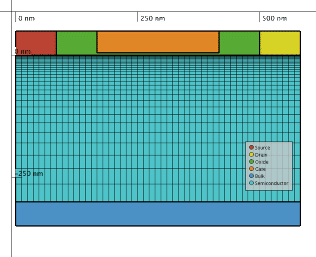(a)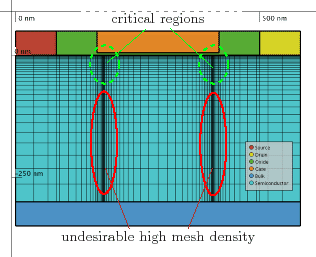(b)

An additional disadvantage of these structured grids becomes significant for devices with non-planar surfaces. The inflexibility of this mesh type does not permit a smooth representation of non-planar surfaces. An example of such a stepped geometry near a birds beak in an LDMOS transistor is shown in Fig. 7.2(a).

Figure 7.2: Representation of non-planar surfaces using orthogonal grids (a) and triangular grids (b).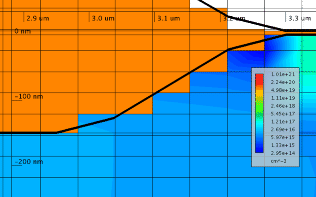(a) Orthogonal grid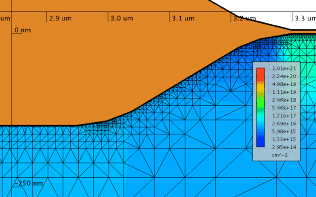(b) Triangular grid

Utilizing unstructured meshes overcomes the disadvantages mentioned above. The mesh is based on triangular elements in two dimensions or tetrahedral elements in three dimensions. In Fig. 7.2(b) and 7.3 sample meshes are shown. These meshes are often based on structured meshes with refinements in areas of special interest. However, generation of unstructured meshes suitable for device simulation is a cumbersome task, especially in three-dimensional devices .

Figure 7.3: The mesh of the sample device is based on an orthogonal grid which is triangulated and refined. Areas of special interest are the channel and the junction regions which thus require a denser mesh (a). A three-dimensional sample mesh of the channel area of a MOS device (b).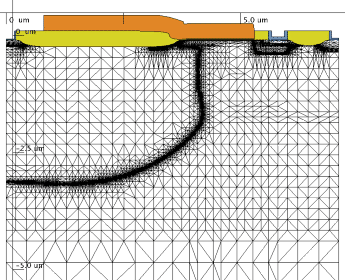(a) Two-Dimensional Mesh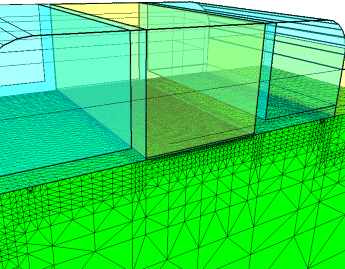(b) Three-Dimensional Mesh

The box discretization introduced in the next section requires the mesh to fulfill the Delaunay criterion . This criterion describes unstructured meshes constructed for a set of vertices such that no vertex lies inside the circumscribed circle of any triangle (see Fig. 7.4) or the circumscribed sphere of any tetrahedron. This is always valid for triangulated rectangular grids but can be difficult to guarantee in general.

Figure 7.4: A Delaunay mesh (a) and its Voronoi tessellation (b), also called the dual mesh, is shown (created using ). The circumscribed circles of three randomly selected triangles are emphasized. Every mesh point has an associated volume - every point in the domain has exactly one associated box volume.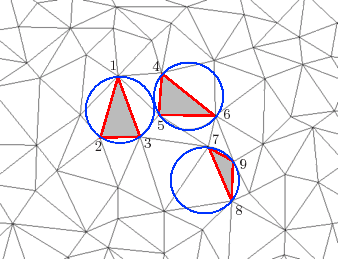(a) Delaunay Mesh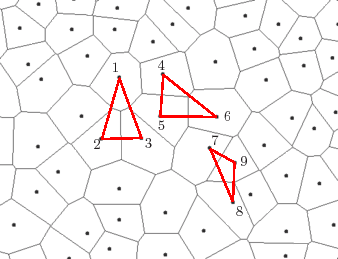(b) Voronoi Tessellation

Other obstacles during mesh generation are the proper representation of borders and surfaces and the definition of mesh refinement criteria for areas of special interest which require higher resolutions. The details of the mesh refinement procedure often have to be specified manually, since mesh generation tools have no a-priori information of the device behavior. A typical refinement strategy is performed in the dependence of the gradient of the net dopant concentration which is equivalent to the built-in potential. This provides a fine mesh at the metallurgic pn-junctions. One has to consider that depending on the bias of the device in operation, the carrier concentrations can change so that the effective junctions and therefore the positions of the highest gradients of the electrostatic potential and carrier concentrations move. Also the current flow depends on the bias of the system and the direction of currents and fields can change. Special refinement in the channel areas in MOS transistors can be specified using rules which depend on the distance to the gate oxide segment. Depending on the simulations to be performed, a refinement in the gate oxide segments is required. Since fully automatic mesh generation would clearly simplify the work-flow, considerable efforts have been put into the development of methods for automatic mesh generation. A promising approach applies error estimation algorithms on simulation results . This information is recursively used to optimize the simulation mesh.Next: 7.2 Box Discretization Up: 7. Numerical Considerations Previous: 7. Numerical Considerations

O. Triebl: Reliability Issues in High-Voltage Semiconductor Devices